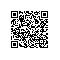# 通过从零开始实现一个感知机模型，我学到了这些

什么是二元分类器（Binary Classifier）？## 怎么搭建机器学习模型？

1. 预处理

2. 训练

3. 评估

4. 预测## 实现感知机

self.w_ = np.zeros(1 + X.shape)

for _ in range(self.n_iter):

self.w_ = np.zeros(1 + X.shape)
for _ in range(self.n_iter):
for xi, target in zip(X, y):
update = self.eta * (target - self.predict(xi))
self.w_[1:] += update * xi
self.w_ += update
def net_input(self, X):
"""Calculate net input"""
return np.dot(X, self.w_[1:]) + self.w_
def predict(self, X):
"""Return class label after unit step"""
return np.where(self.net_input(X) >= 0.0, 1, -1)## 收获1：参数的理解

# Partial portion of the "fit" function
for xi, target in zip(X, y):
update = self.eta * (target - self.predict(xi))
self.w_[1:] += update * xi
self.w_ += update
errors += int(update != 0.0)

for _ in range(self.n_iter):
errors = 0
for xi, target in zip(X, y):
update = self.eta * (target - self.predict(xi))
self.w_[1:] += update * xi
self.w_ += update
errors += int(update != 0.0)
self.errors_.append(errors)

## 收获3：一种通用的学习方法